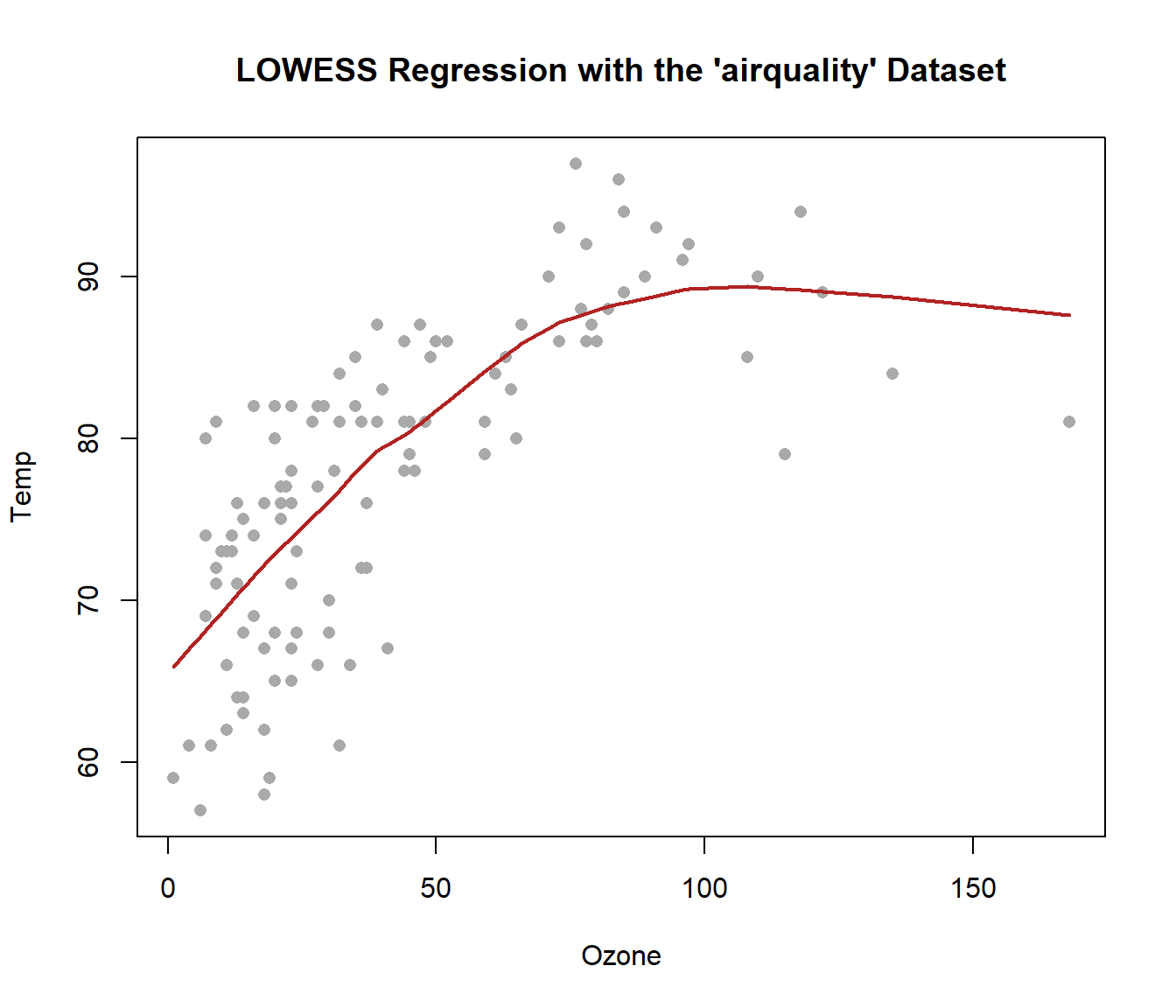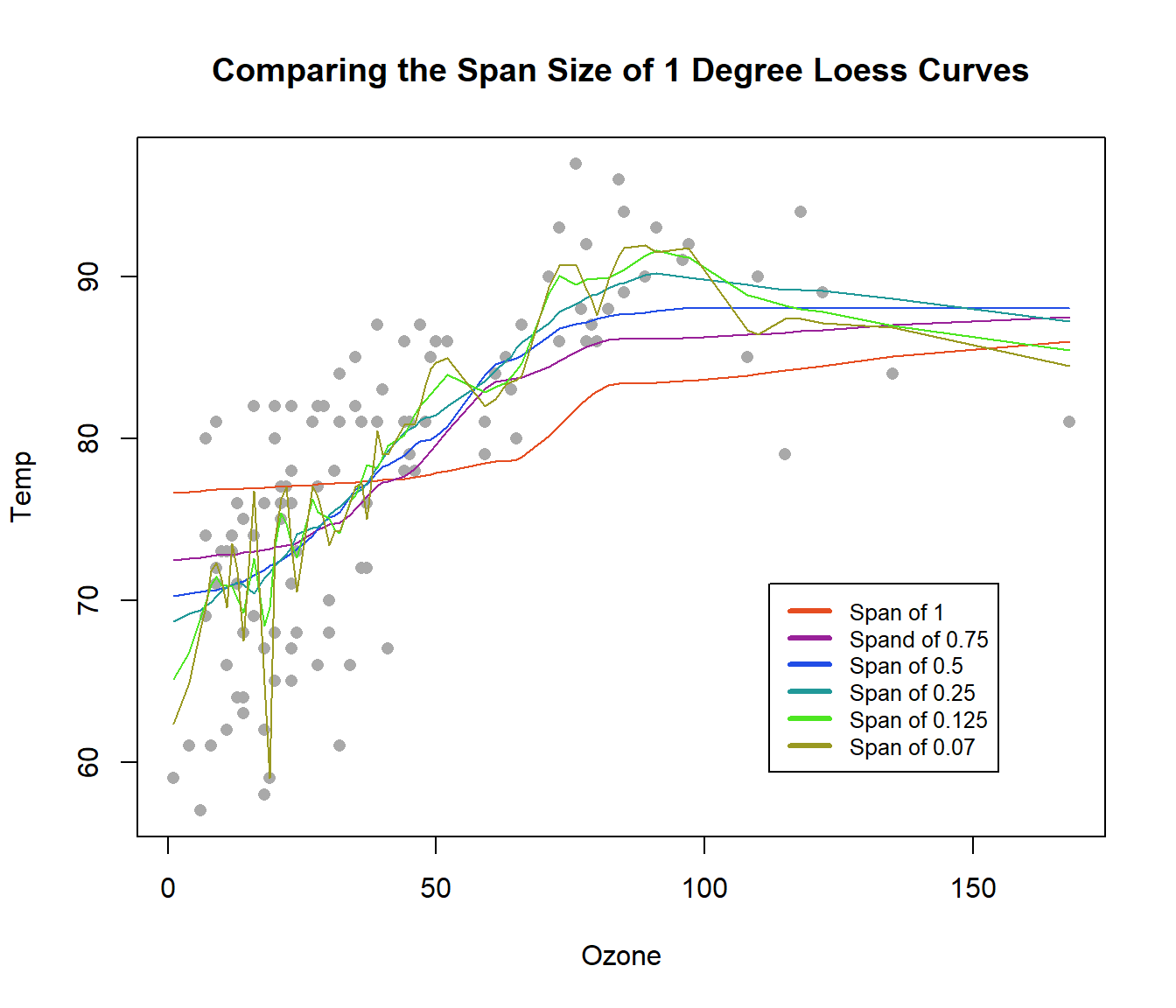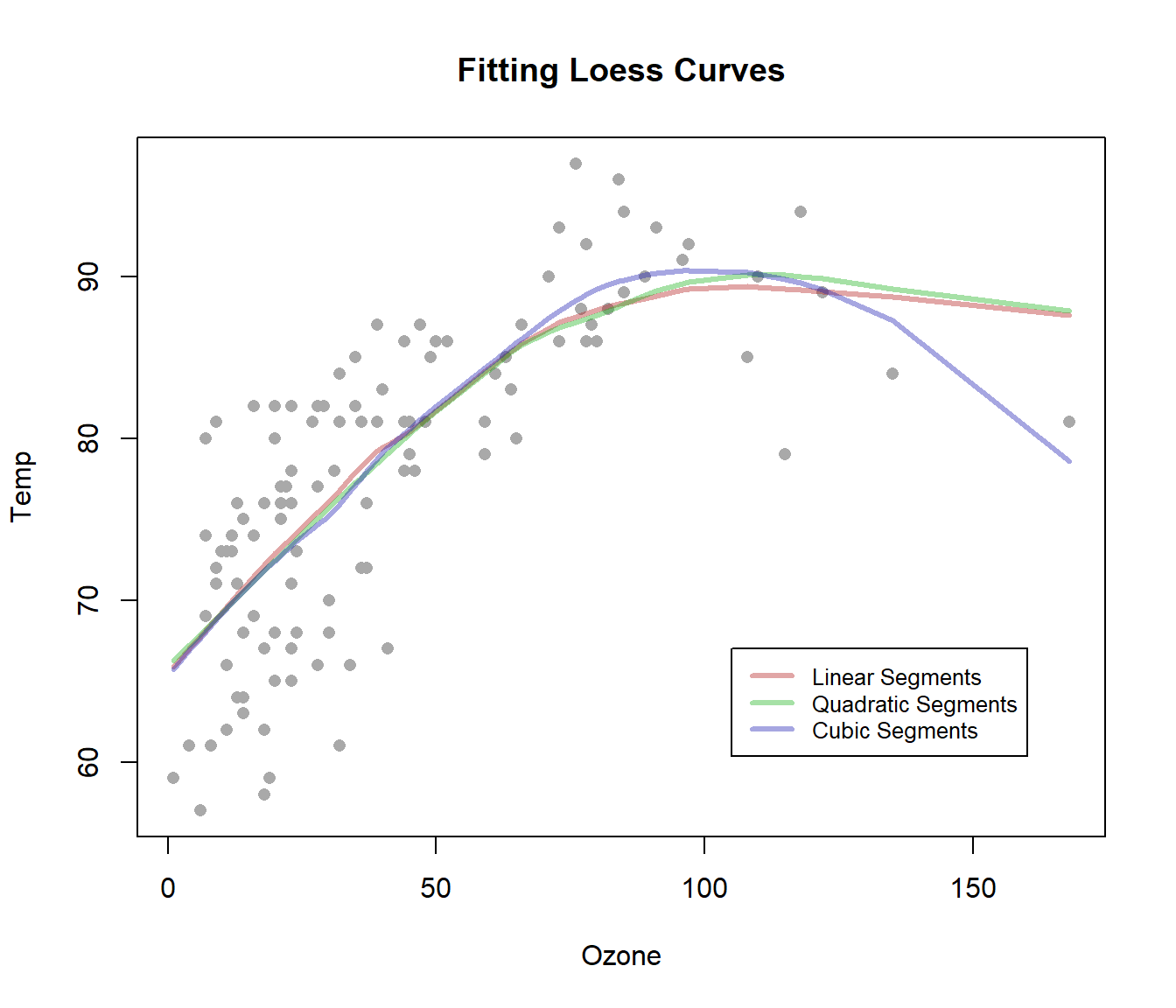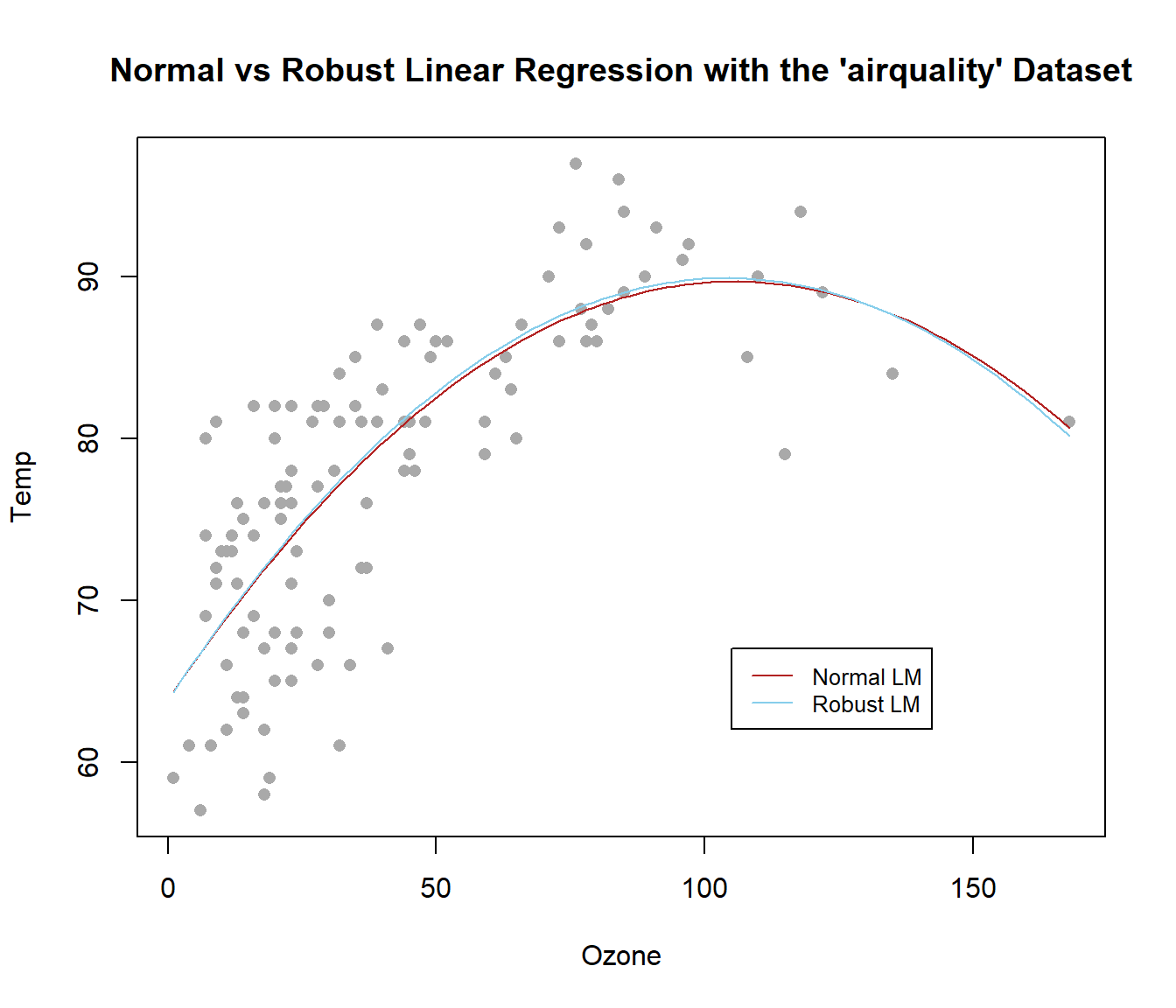I’ll be using these libraries:

``````library(tidyverse)
library(MASS)
library(carData)``````

## LOWESS Curves

The LOWESS and LOESS functions create a curve by joining a bunch of localized regression lines together.

``````# Note: This plot was taken from Garrett Saunders' notebook:
# See https://byuistats.github.io/Statistics-Notebook/LinearRegression.html; it contains great information.

# By selecting just Temp and Ozone before removing the NAs, it yeilds a fuller dataset.
air2 <- na.omit(dplyr::select(airquality, Temp, Ozone))

plot(Temp ~ Ozone, data = air2, pch = 16, col = "darkgray", main = "LOWESS Regression with the 'airquality' Dataset")

lowess_air <- lowess(air2\$Ozone, air2\$Temp)
lines(lowess_air, col = "firebrick", lwd = 2)``````Although they provide no interpretable statistical function, LOWESS and LOESS both create a model that can be used to predict new y values.

``````loess_air <- loess(Temp ~ Ozone, data = air2)
predict(loess_air, data.frame(Ozone = 40))``````

A word of caution: You should rarely use Lowess to predict y values for anything outside the domain of the data you created the model from.

If you load library(MASS) it will mask the select() function from the dplyr package and you won’t be able to use it.

There are several solutions to this problem (Google “r prevent masking”), but here are two:

#### 1

Run

``unloadNamespace("MASS")``

Then run

``library(MASS)``

when you need it.

#### 2

Just use

``dplyr::select()``

when you need to use select().

LOWESS and LOESS are very similar, but they have different default parameters and LOESS allows for more than one explanatory variable.

LOESS stands for “locally estimated scatterplot smoothing” and LOWESS for “locally weighted regression and smoothing scatterplots”.

To generate each regression line, the x (or explanatory) values nearest to the current x are included and then given a weight for their influence the final least squares regression. The purpose is simply to choose the best y (response) value. The process for finding each localized regression is iterative: A weighted regression is performed for each neighborhood of points and then the weighting of the points is updated and another regression is performed, until a certain stopping criterion. The curve seen is a connecting of all the invisible dots that denote the ‘best’ fit y values.

The x values included in each localized regression are determined by the span of the lowess function. The span is a percentage of all the data points.

``````plot(Temp ~ Ozone, data = air2, pch = 16, col = "darkgray", main = "Comparing the Span Size of 1 Degree Loess Curves")

# Solves a problem with the loess() function: The lines will be connected in the right order.
air2 <- arrange(air2, desc(Ozone))

# Add degree 1 loess with span of 1
lo_s1 <- loess(Temp ~ Ozone, data = air2, degree = 0, span = 1)
lines(lo_s1\$fit ~ Ozone, data = air2, col = rgb(red = .9, green = .3, blue = .13), lwd = 1)

# Add degree 1 loess with span of 0.75
lo_s2 <- loess(Temp ~ Ozone, data = air2, degree = 0, span = 0.75)
lines(lo_s2\$fit ~ Ozone, data = air2, col = rgb(red = .6, green = .13, blue = .6), lwd = 1)

# Add degree 1 loess with span of 0.5
lo_s3 <- loess(Temp ~ Ozone, data = air2, degree = 0, span = 0.5)
lines(lo_s3\$fit ~ Ozone, data = air2, col = rgb(red = .13, green = .3, blue = .9), lwd = 1)

# Add degree 1 loess with span of 0.25
lo_s4 <- loess(Temp ~ Ozone, data = air2, degree = 0, span = 0.25)
lines(lo_s4\$fit ~ Ozone, data = air2, col = rgb(red = .13, green = .6, blue = .6), lwd = 1)

# Add degree 1 loess with span of 0.125
lo_s5 <- loess(Temp ~ Ozone, data = air2, degree = 0, span = 0.125)
lines(lo_s5\$fit ~ Ozone, data = air2, col = rgb(red = .3, green = .9, blue = .13), lwd = 1)

# Add degree 1 loess with span of 0.07
lo_s6 <- loess(Temp ~ Ozone, data = air2, degree = 0, span = 0.07)
lines(lo_s6\$fit ~ Ozone, data = air2, col = rgb(red = .6, green = .6, blue = .13), lwd = 1)

legend(112, 71, legend = c("Span of 1", "Spand of 0.75", "Span of 0.5", "Span of 0.25", "Span of 0.125", "Span of 0.07"), col = c(rgb(red = .9, green = .3, blue = .13), rgb(red = .6, green = .13, blue = .6), rgb(red = .13, green = .3, blue = .9), rgb(red = .13, green = .6, blue = .6), rgb(red = .3, green = .9, blue = .13), rgb(red = .6, green = .6, blue = .13)), lwd = 3, cex = 0.8)``````There is also a degree parameter, which if increased results in connecting the dots via parabolas, cubic curves, and so on. See https://en.wikipedia.org/wiki/Degree_of_a_polynomial.

``````plot(Temp ~ Ozone, data = air2, pch = 16, col = "darkgray", main = "Fitting Loess Curves")
lines(lowess(air2\$Ozone, air2\$Temp), col = rgb(red = .7, green = .13, blue = .13, alpha = 0.4), lwd = 3)

lo_d2 <- loess(Temp ~ Ozone, data = air2, degree = 1)
lines(lo_d2\$fit ~ Ozone, data = air2, col = rgb(red = .13, green = .7, blue = .13, alpha = 0.4), lwd = 3)

# Add curve with cubic segments
lo_d3 <- loess(Temp ~ Ozone, data = air2, degree = 2)
lines(lo_d3\$fit ~ Ozone, data = air2, col = rgb(red = .13, green = .13, blue = .7, alpha = 0.4), lwd = 3)

legend(105, 67, legend = c("Linear Segments", "Quadratic Segments", "Cubic Segments"),
col = c(rgb(red = .7, green = .13, blue = .13, alpha = 0.4), rgb(red = .13, green = .7, blue = .13, alpha = 0.4), rgb(red = .13, green = .13, blue = .7, alpha = 0.4)), lwd = 3, cex = 0.8)``````## Robust Linear Models

A robust linear model can be called the same way as a normal linear model:

``````air_lm <- lm(Temp ~ Ozone + I(Ozone^2), air2)
air_rlm <- rlm(Temp ~ Ozone + I(Ozone^2), air2)
summary(air_lm)
summary(air_rlm)``````
[SHOW OUTPUT]

The difference with a robust linear model is that while the typical linear regression assumes all data is equally important, robust models reduce the weight of points depending on how much the line changes if those points are removed. The points that would influence the line more are trusted less. It is also an iterative process: A normal initial regression is done and then the weights are computed. Then a new regression is done, and so on, until the model stops changing.

``````plot(Temp ~ Ozone, air2, pch = 16, col = "darkgray", main = "Normal vs Robust Linear Regression with the 'airquality' Dataset")
curve(air_lm\$coef + air_lm\$coef*x + air_lm\$coef*x^2, add = T, col = "firebrick")
curve(air_rlm\$coef + air_rlm\$coef*x + air_rlm\$coef*x^2, add = T, col = "skyblue")
legend(105, 67, legend=c("Normal LM", "Robust LM"),
col=c("firebrick", "skyblue"), lwd = 1, cex = 0.8)``````In the previous plot, the robust and traditional regression are very similar, but depending on the model you are trying to fit and the shape of the data, they can be very different:

``````plot(weight ~ height, Davis, pch = 16, col = "darkgray", main = "Normal vs Robust Linear Regression with 'Davis' the Dataset")

davis_lm <- lm(weight ~ I(height^2), data = Davis)
davis_rlm <- rlm(weight ~ I(height^2), data = Davis)

curve(davis_lm\$coef + davis_lm\$coef*x^2, add = T, col = "firebrick", lwd = 2)
curve(davis_rlm\$coef + davis_rlm\$coef*x^2, add = T, col = "skyblue", lwd = 2)
legend(95, 78, legend = c("Normal LM", "Robust LM"),
col = c("firebrick", "skyblue"), lwd = 2, cex = 0.8)``````